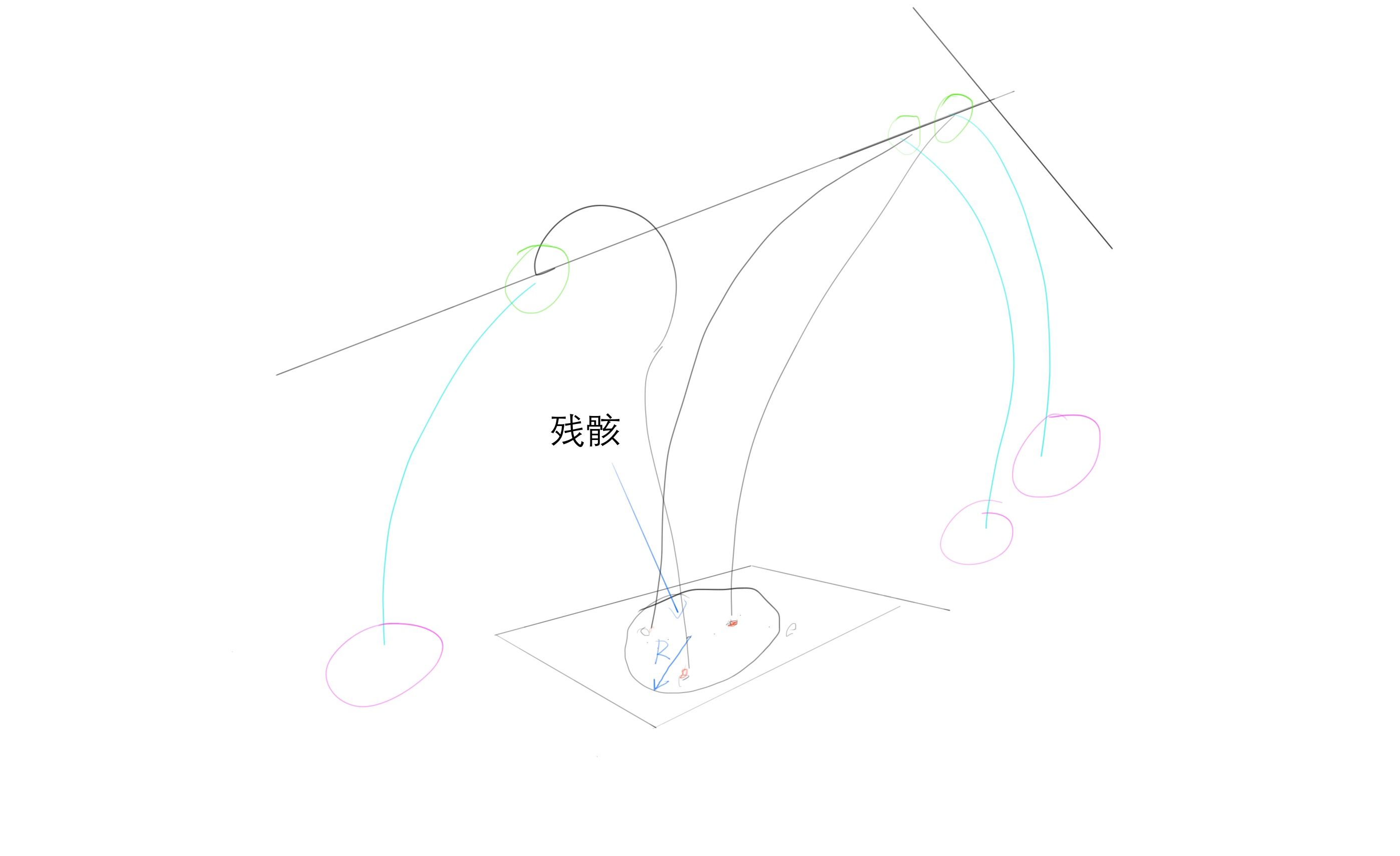# 数学建模

The utilization of the 搜查机 would reach maximization if we can establish a rational 搜查方案。我们的思路是将这个问题分为四个步骤，1、建立飞机坠毁地点的概率模型。2、建立搜索模型。3、用计算机模拟规划路线进行飞行器搜素。4.将飞行器搜索的反馈结果作为依据，更新我们的概率模型

## 失恋后飞行器的概率估计

$$!\int \psi(x,y,z,t)dx dy dz = 1$$

• 生成一堆粒子，产生于飞行器失联点，位置，速度，高度，航向与原飞行器保持一致
• 对粒子进行跌代，迭代过程中粒子包括三个状态：飞行，坠落，漂流。
1. 如果粒子处于飞行状态，以一定的概率发生转向，另外以一定的概率爆炸坠落。
2.如果粒子处于坠落状态，受到力运动，加速方程由下面的受力分析给出，直到落到洋面转化为漂流状态
3.如果粒子处于漂流状态，则随洋面漂移，漂移的速度这里我们简化为匀速，如果有足够的时间可以引入来自 http://www.ccpo.odu.edu/POMWEB/userinfo.htm的数据

-迭代一定时间后可以获得粒子基本上都坠落入海洋

## 搜寻算法

### 蚁群算法

$$!P^{ka}_{i,j}= (Info_{full}-Info^b) \cdot 100; if (Info^b>0)$$
$$!P^{ka}_{i,j}= value (2 \cdot Info_{full}-(Info_b+max(Info_{other}))) ;if (Info^b=0)$$

$$!P^{kb}_{i,j}= (Info_{full}-Info^b) \cdot 100; if (Info^a>0)$$
$$!P^{kb}_{i,j}= value (2 \cdot Info_{full}-(Info_b+max(Info_{other}))) ;if (Info^a=0)$$

### 另一个问题，如何根据一片残骸找到更多的残骸## “数学建模”上的1条回复liu说：